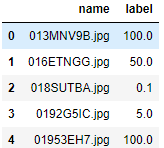# Label_from_df can't identify the labels

Hi, I try to build a classifier and the type of label is float. It is shown as bellows:My code is as follows:
src = (ImageList.from_df(df, TRN, cols=‘name’)
.split_by_rand_pct())
data = (src.label_from_df(cols=’ label’, classes=classes)
.transform(size=300)
.databunch(bs=4)
.normalize(imagenet_stats))
It seems the ‘label_from_df’ could not identify the labels, because I find the data.c is always 1.
How should I solve the problem?

You labels are floats, so the fastai library interpreted them as such. In that case `data.c` is set to 1 as you want only one output for your model (that float) and the loss function that will be automatically picked for you is the mean-squared error.

Thank! I see. If the type of the labels is float, the task is regarded as a regression task, so the data.c is 1.